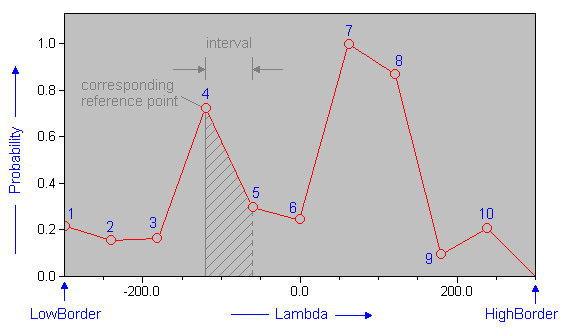The SDL Component Suite is an industry leading collection of components supporting scientific and engineering computing. Please visit the SDL Web site for more information....## Probability

 Unit: SDL_statis Class: Declaration: property Probability[ix: integer]: double;

The array property Probability defines the evenly distributed reference points for the probability distribution function. The parameter ix runs from 1 to Resolution and is an index into the Probability array. The reference points are located at the left (lower) side of each interval. The probability within one interval is interpolated between its reference point and the reference point of the next interval. The probability of the last interval is extrapolated towards zero.After changing the Probability property, the method Normalize should be called in order to ensure that the probabilities are scaled to values between 0.0 and 1.0.

 Hint: The array property Probability does not form some kind of probability density function. The maximum value of all entries in this array should always equal 1.0.

Last Update: 2012-Oct-20